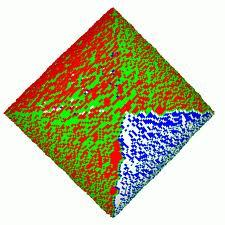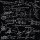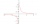# Rhombus and inscribed circle

It is given a rhombus with side a = 6 cm and the radius of the inscribed circle r = 2 cm. Calculate the length of its two diagonals.

Result

u =  11.21 cm
v =  4.282 cm

#### Solution:

$a=6 \ \text{cm} \ \\ r=2 \ \text{cm} \ \\ \ \\ a=a_{1}+a_{2} \ \\ r^2=a_{1}a_{2} \ \\ a_{1}(a-a_{1})=r^2 \ \\ \ \\ \ \\ x(6-x)=2^2 \ \\ -x^2 +6x -4=0 \ \\ x^2 -6x +4=0 \ \\ \ \\ a=1; b=-6; c=4 \ \\ D=b^2 - 4ac=6^2 - 4\cdot 1 \cdot 4=20 \ \\ D>0 \ \\ \ \\ x_{1,2}=\dfrac{ -b \pm \sqrt{ D } }{ 2a }=\dfrac{ 6 \pm \sqrt{ 20 } }{ 2 }=\dfrac{ 6 \pm 2 \sqrt{ 5 } }{ 2 } \ \\ x_{1,2}=3 \pm 2.2360679774998 \ \\ x_{1}=5.2360679774998 \ \\ x_{2}=0.76393202250021 \ \\ \ \\ \text{ Factored form of the equation: } \ \\ (x -5.2360679774998) (x -0.76393202250021)=0 \ \\ \ \\ a_{1}=x_{1}=5.2361 \doteq 5.2361 \ \\ a_{2}=x_{2}=0.7639 \doteq 0.7639 \ \\ \ \\ (u/2)^2=a_{1}^2 + r^2 \ \\ u=2 \cdot \ \sqrt{ a_{1}^2 + r^2 }=2 \cdot \ \sqrt{ 5.2361^2 + 2^2 } \doteq 11.2101 \doteq 11.21 \ \text{cm}$

Checkout calculation with our calculator of quadratic equations.

$(v/2)^2=a_{2}^2 + r^2 \ \\ v=2 \cdot \ \sqrt{ a_{2}^2 + r^2 }=2 \cdot \ \sqrt{ 0.7639^2 + 2^2 } \doteq 4.2819 \doteq 4.282 \ \text{cm} \ \\ \ \\ r_{2}=\sqrt{ a_{1} \cdot \ a_{2} }=\sqrt{ 5.2361 \cdot \ 0.7639 }=2 \ \\ r_{2}=r$Our examples were largely sent or created by pupils and students themselves. Therefore, we would be pleased if you could send us any errors you found, spelling mistakes, or rephasing the example. Thank you!

Leave us a comment of this math problem and its solution (i.e. if it is still somewhat unclear...):

Showing 1 comment:Math student
The diagonal of a rhombus measure 16cm and 30cm find its perimeterTips to related online calculators
Looking for help with calculating roots of a quadratic equation?
Pythagorean theorem is the base for the right triangle calculator.

## Next similar math problems:Find the radius of the circle with area S = 200 cm².
2. FlowerbedWe enlarge the circular flower bed, so its radius increased by 3 m. The substrate consumption per enlarged flower bed was (at the same layer height as before magnification) nine times greater than before. Determine the original flowerbed radius.
3. SupposeSuppose you know that the length of a line segment is 15, x2=6, y2=14 and x1= -3. Find the possible value of y1. Is there more than one possible answer? Why or why not?
4. Medians in right triangleIt is given a right triangle, angle C is 90 degrees. I know it medians t1 = 8 cm and median t2 = 12 cm. .. How to calculate the length of the sides?
5. Secret treasureScouts have a tent in the shape of a regular quadrilateral pyramid with a side of the base 4 m and a height of 3 m. Determine the radius r (and height h) of the container so that they can hide the largest possible treasure.
6. Square sideIf we enlarge the square side a = 5m, its area will increase by 10,25%. How many percent will the side of the square increase? How many percent will it increase the circumference of the square?
7. Rectangle fieldThe field has a shape of a rectangle having a length of 119 m and a width of 19 m. , How many meters have to shorten its length and increase its width to maintain its area and circumference increased by 24 m?
8. A rectangle 2A rectangle has a diagonal length of 74cm. Its side lengths are in ratio 5:3. Find its side lengths.
9. Sides of right angled triangleOne leg is 1 m shorter than the hypotenuse, and the second leg is 2 m shorter than the hypotenuse. Find the lengths of all sides of the right-angled triangle.
10. Block or cuboidThe wall diagonals of the block have sizes of √29cm, √34cm, √13cm. Calculate the surface and volume of the block.
11. Three parallelsThe vertices of an equilateral triangle lie on 3 different parallel lines. The middle line is 5 m and 3 m distant from the end lines. Calculate the height of this triangle.
12. The touristThe tourist wanted to walk the route 16 km at a specific time. He, therefore, came out at the necessary constant speed. After a 4 km walk, however, he fell unplanned into the lake, where he almost drowned. It took him 20 minutes to get to the shore and re
13. TV competitionIn the competition, 10 contestants answer five questions, one question per round. Anyone who answers correctly will receive as many points as the number of competitors answered incorrectly in that round. One of the contestants after the contest said: We
14. Conical bottleWhen a conical bottle rests on its flat base, the water in the bottle is 8 cm from it vertex. When the same conical bottle is turned upside down, the water level is 2 cm from its base. What is the height of the bottle?
15. The productThe product of a number plus that number and its inverse is two and one-half. What is the inverse of this number
16. Before yesterdayHe merchant adds a sale sign in his shop window to the showed pair of shoes in the morning: "Today by p% cheaper than yesterday. " After a while, however, he decided that the sign saying: "Today 62.5% cheaper than the day before yesterday". Determine the
17. Equation 23Find value of unknown x in equation: x+3/x+1=5 (problem finding x)